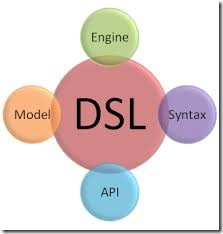0
0这是《从Java和JavaScript来学习Haskell和Groovy》系列的第四篇。

DSL（Domain Specific Language）指的是一定应用领域内的计算机语言，它可以不强大，它可以只能在一定的领域内生效（和GPL相比，GPL是General Purpose Language），表达仅限于该领域，但是它对于特定领域简洁、清晰，包含针对特定领域的优化。

```document
.html()
.body()
.p()
.text("context 1")
.end()
.p()
.text("context 2")
.end()
.end()
.end()
.creat();```

```new Map(
city("Beijing", x1, y1),
city("Shanghai", x2, y2),
city("Guangzhou", x3, y3)
);```

```public class T {
public Integer val;
}```

```Collections.sort(list, new Comparator<T>() {
@Override
public int compare(T o1, T o2) {
return o1.val.compareTo(o2.val);
}
});```

`Collections.sort(list, (x, y) -> y - x);`

```@FunctionalInterface
public interface Comparator<T> {
...
}```

```int compare(T o1, T o2);
boolean equals(Object obj);```

```new Wrapper([1, 2, 5, 3, 4])
.filter(filterFunc)
.map(mapFunc)
.sort()
.zipWith([7, 8, 9, 10, 11]);```

```var logic = new Logic()
.whenTrue(exp1)
.whenFalse(exp2);

console.log(logic.test(3>2));```

```// equivalent to: take(2.pills).of(chloroquinine).after(6.hours)
take 2.pills of chloroquinine after 6.hours```

```show = { println it }
square_root = { Math.sqrt(it) }

[the: { what ->
[of: { n -> action(what(n)) }]
}]
}

please show the square_root of 100
// ==> 10.0```

```def page = new MarkupBuilder()
page.html {
body {
a ( href:'http://...' ) { 'this is a link' }
}
}```

```JsonBuilder builder = new JsonBuilder()
builder.records {
car {
name 'HSV Maloo'
make 'Holden'
year 2006
country 'Australia'
record {
type 'speed'
description 'production pickup truck with speed of 271kph'
}
}
}
String json = JsonOutput.prettyPrint(builder.toString())```

```quicksort :: (Ord a) => [a] -> [a]
quicksort [] = []
quicksort (x:xs) =
let smallerSorted = quicksort [a | a <- xs, a <= x]
biggerSorted = quicksort [a | a <- xs, a > x]
in smallerSorted ++ [x] ++ biggerSorted```

```Prelude> :t max
max :: Ord a => a -> a -> a```

```max 1 2
(max 1) 2```

```len :: (Num b) => [a] -> b
len [] = 0
len (_:xs) = 1 + len xs```

`sum (takeWhile (<10) (filter odd (map (^2) [1..])))`

```sum . takeWhile (<10) . filter odd . map (^2) \$ [1..]
```